# 1320 Christmas Factor Trees

### Today’s Puzzle:

Regardless of its size, an evergreen tree is a mighty symbol at Christmastime. Today’s factoring puzzle features a couple of relatively small Christmas trees, but don’t think for even one minute that these little trees make for an easy puzzle. It’s a level 6 puzzle so there are several places that the clues might trick you. Use logic throughout the entire process, and you should be able to solve it!

Print the puzzles or type the solution in this excel file: 12 factors 1311-1319

### Factor Trees for 1320:

That factoring puzzle has nothing to do with the factors of 1320. In case you are looking for factor trees for the number 1320, here are a few of the MANY possible ones:

### Factors of 1320:

• 1320 is a composite number.
• Prime factorization: 1320 = 2 × 2 × 2 × 3 × 5 × 11, which can be written 1320 = 2³ × 3 × 5 × 11.
• The exponents in the prime factorization are 3, 1, 1, and 1. Adding one to each and multiplying we get (3 + 1)(1 + 1)(1 + 1) )(1 + 1) = 4 × 2 × 2 × 2 = 32. Therefore 1320 has exactly 32 factors.
• Factors of 1320: 1, 2, 3, 4, 5, 6, 8, 10, 11, 12, 15, 20, 22, 24, 30, 33, 40, 44, 55, 60, 66, 88, 110, 120, 132, 165, 220, 264, 330, 440, 660, 1320
• Factor pairs: 1320 = 1 × 1320, 2 × 660, 3 × 440, 4 × 330, 5 × 264, 6 × 220, 8 × 165, 10 × 132, 11 × 120, 12 × 110, 15 × 88, 20 × 66, 22 × 60, 24 × 55, 30 × 44 or 33 × 40
• Taking the factor pair with the largest square number factor, we get √1320 = (√4)(√330) = 2√330 ≈ 36.331804

### Sum-Difference Puzzles:

330 has eight factor pairs. One of those pairs adds up to 61, and  another one subtracts to 61. Put the factors in the appropriate boxes in the first puzzle.

1320 has sixteen factor pairs. One of the factor pairs adds up to ­122, and a different one subtracts to 122. If you can identify those factor pairs, then you can solve the second puzzle!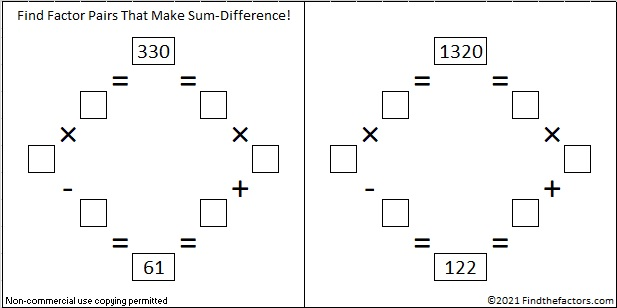The second puzzle is really just the first puzzle in disguise. Why would I say that?

1320 is the third number that is at the top of more than one Sum-Difference Puzzle. This next one is a primitive.

Again, 1320 has sixteen factor pairs. One of the factor pairs adds up to 73, and a different one subtracts to 73. If you can identify those factor pairs, then you can solve this puzzle!If you would like a little help finding those factor pairs that make sum-difference, the chart below lists all of 1320’s factor pairs with their sums and their differences.### More about the Number 1320:

1320 is the sum of consecutive primes FOUR different ways:
It is the sum of the eighteen primes from 37 to 109,
107 + 109 + 113 + 127 + 131 + 137 + 139 + 149 + 151 + 157 = 1320,
149 + 151 + 157 + 163 + 167 + 173 + 179 + 181 = 1320, and
659 + 661 = 1320.

1320 is the hypotenuse of a Pythagorean triple:
792-1056-1320 which is (3-4-5) times 264.

# 326 Tiny Christmas Factor Tree

• 326 is a composite number.
• Prime factorization: 326 = 2 x 163
• The exponents in the prime factorization are 1 and 1. Adding one to each and multiplying we get (1 + 1)(1 + 1) = 2 x 2 = 4. Therefore 326 has exactly 4 factors.
• Factors of 326: 1, 2, 163, 326
• Factor pairs: 326 = 1 x 326 or 2 x 163
• 326 has no square factors that allow its square root to be simplified. √326 ≈ 18.055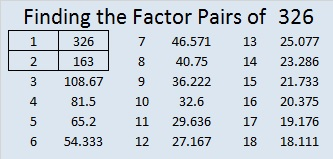Even though 326 is a three digit number, there is only one way to construct its factor tree, two if you count its mirror image. Either way is illustrated here. Below them is a Christmas factor tree puzzle that is a lot more interesting than the factor tree for 326.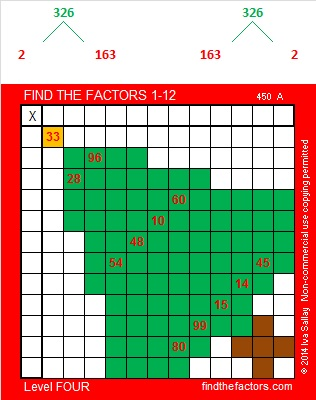Print the puzzles or type the factors on this excel file: 12 Factors 2014-12-15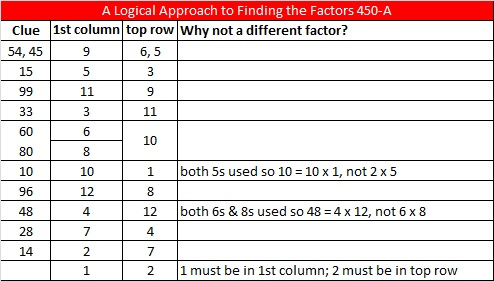# 324 Christmas Factor Trees

• 324 is a composite number.
• Prime factorization: 324 = 2 x 2 x 3 x 3 x 3 x 3, which can be written 324 = (2^2) x (3^4)
• The exponents in the prime factorization are 2 and 4. Adding one to each and multiplying we get (2 + 1)(4 + 1) = 3 x 5 = 15. Therefore 324 has exactly 15 factors.
• Factors of 324: 1, 2, 3, 4, 6, 9, 12, 18, 27, 36, 54, 81, 108, 162, 324
• Factor pairs: 324 = 1 x 324, 2 x 162, 3 x 108, 4 x 81, 6 x 54, 9 x 36, 12 x 27, or 18 x 18
• 324 is a perfect square. √324 = 18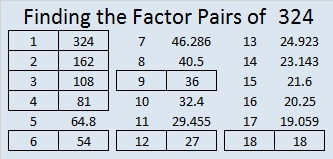Included in these 324 factor trees are smaller factor trees for the numbers in brown: 4, 6, 9, 12, 18, 27, 36, 54, 81, 108, and 162. Prime factors of 324 are in red.This Level 2 puzzle looks like part of a Christmas tree branch. It’s not too difficult to solve. Give it a try!Print the puzzles or type the factors on this excel file: 12 Factors 2014-12-15# 320 Christmas Factor Trees

• 320 is a composite number.
• Prime factorization: 320 = 2 x 2 x 2 x 2 x 2 x 2 x 5, which can be written 320 = 2⁶ x 5
• The exponents in the prime factorization are 6, and 1. Adding one to each and multiplying we get (6 + 1)(1 + 1) = 7 x 2 = 14. Therefore 320 has exactly 14 factors.
• Factors of 320: 1, 2, 4, 5, 8, 10, 16, 20, 32, 40, 64, 80, 160, 320
• Factor pairs: 320 = 1 x 320, 2 x 160, 4 x 80, 5 x 64, 8 x 40, 10 x 32, or 16 x 20
• Taking the factor pair with the largest square number factor, we get √320 = (√5)(√64) = 8√5 ≈ 17.889In these factor trees for 320, we can also see factor trees for 4, 8, 10, 16, 20, 32, 40, 64, 80, and 160. The tops of each of those factor trees are in brown. No matter which factor pairs we use to build the tree, we always get the same prime factors which are indicated in red.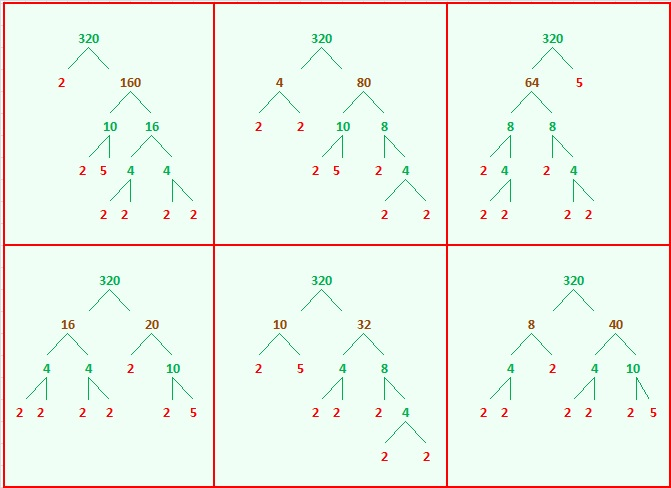Now try finding the factors in this Christmas tree puzzle that make it work like a multiplication table:Print the puzzles or type the factors on this excel file: 10 Factors 2014-12-08

A Logical Approach to FIND THE FACTORS: Find the column or row with two clues and find their common factor. Write the corresponding factors in the factor column (1st column) and factor row (top row).  Because this is a level three puzzle, you have now written a factor at the top of the factor column. Continue to work from the top of the factor column to the bottom, finding factors and filling in the factor column and the factor row one cell at a time as you go.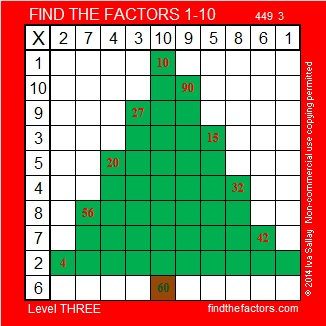# 318 Christmas Factor Tree Gif

• 318 is a composite number.
• Prime factorization: 318 = 2 x 3 x 53
• The exponents in the prime factorization are 1, 1, and 1. Adding one to each and multiplying we get (1 + 1)(1 + 1)(1 + 1) = 2 x 2 x 2 = 8. Therefore 318 has exactly 8 factors.
• Factors of 318: 1, 2, 3, 6, 53, 106, 159, 318
• Factor pairs: 318 = 1 x 318, 2 x 159, 3 x 106, or 6 x 53
• 318 has no square factors that allow its square root to be simplified. √318 ≈ 17.833This factoring tree gif solves and completes this Level ONE puzzle right before your eyes. Scroll down to see three possible factor trees for the number 318.make animated gifs like this at MakeAGif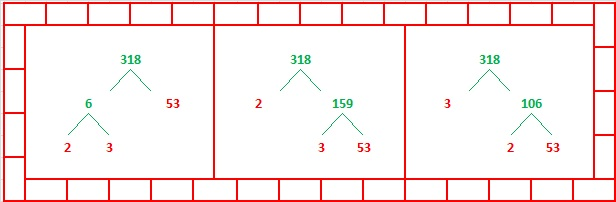Print the puzzles or type the factors on this excel file: 10 Factors 2014-12-08

# 316 Christmas Factor Trees

• 316 is a composite number.
• Prime factorization: 316 = 2 x 2 x 79, which can be written 316 = 2² x 79
• The exponents in the prime factorization are 2 and 1. Adding one to each and multiplying we get (2 + 1)(1 + 1) = 3 x 2  = 6. Therefore 316 has exactly 6 factors.
• Factors of 316: 1, 2, 4, 79, 158, 316
• Factor pairs: 316 = 1 x 316, 2 x 158, or 4 x 79
• Taking the factor pair with the largest square number factor, we get √316 = (√4)(√79) = 2√79 ≈ 17.776Today is all about Christmas trees. You can read how one family set up a beautiful Christmas tree at three-things-thursday-tree-edition, solve the Christmas tree puzzle, and scroll down to see two possible factor trees for 316.

Then polish up your shoes and boots. Place them near your front door or window so they will be ready to be filled with treats when Mikulás (St. Nickolas) makes his rounds tonight.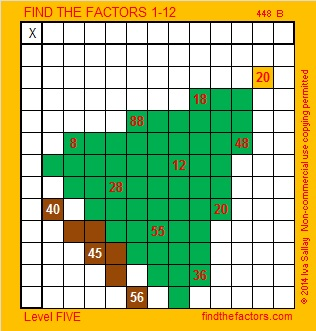Print the puzzles or type the factors on this excel file: 12 Factors 2014-12-01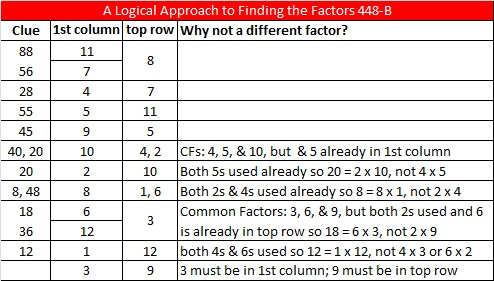# 315 Christmas Factor Trees

315 is the smallest number that is divisible by every 1-digit odd number.

• 315 is a composite number.
• Prime factorization: 315 = 3 × 3 × 5 × 7, which can be written 315 = 3² × 5 × 7
• The exponents in the prime factorization are 2, 1, and 1. Adding one to each and multiplying we get (2 + 1)(1 + 1)(1 + 1) = 3 x 2 x 2 = 12. Therefore 315 has exactly 12 factors.
• Factors of 315: 1, 3, 5, 7, 9, 15, 21, 35, 45, 63, 105, 315
• Factor pairs: 315 = 1 x 315, 3 x 105, 5 x 63, 7 x 45, 9 x 35, or 15 x 21
• Taking the factor pair with the largest square number factor, we get √315 = (√9)(√35) = 3√35 ≈ 17.748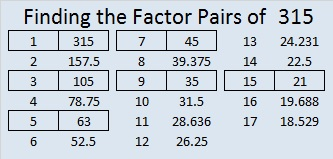Five of the six factor pairs for 315 make lovely Christmas factor trees with the prime factors in red:Here’s a level 4 puzzle that is different kind of Christmas factor tree. Can you complete this mixed-up multiplication table when you’re given only these twelve clues?Print the puzzles or type the factors on this excel file: 12 Factors 2014-12-01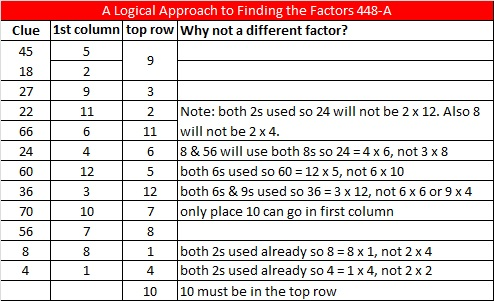# 15 is the Magic Sum of a 3 x 3 Magic Square

15 is a composite number. 15 = 1 x 15 or 3 x 5. Factors of 15: 1, 3, 5, 15. Prime factorization: 15 = 3 x 5.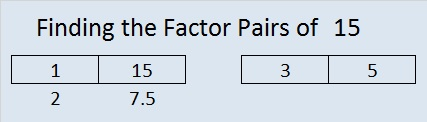When 15 is a clue in the FIND THE FACTORS 1 – 10 or 1 – 12 puzzles, use 3 and 5 as the factors.

If you added the first nine counting numbers together, what sum would you get? What is 1 + 2 +3 + 4+ 5 + 6 + 7 + 8 + 9?

Would you get the same answer by adding (1 + 9) + (2 + 8) + (3 +7) + (4 + 6) + 5?

These are two of the many fun questions you can explore when you try to make a magic square. What is a magic square? If you can place the numbers from 1 to 9 in the box below so that the sum of any row, column, or diagonal will equal the sum of any other row, column, or diagonal, then you will have made a 3 x 3 magic square. The sum of a row, column, or diagonal in a magic square is called the magic sum.Clearly it is not a magic square yet. In fact, only one of the numbers is positioned where it needs to be. Which number do you think is already in the correct position?

When it becomes a magic square, what will the magic sum be? One student noticed that in its current state the sums of the rows are 6, 15, and 24. The sums of the columns are 12, 15, 18. The sums of the diagonals are 15 and 15. Since 15 occurs most often, could the magic sum be 15? One way to determine what the magic sum should be is to add the sums of all three rows and then divide by the number of rows. Since 1 + 2 + 3 + 4 + 5 + 6 + 7 + 8 + 9 = 45 and 45 ÷ 3 = 15, then 15 is indeed the magic sum.

Here are a few easy-to-remember steps to construct a 3 x 3 magic square quickly.

Step 1: Draw a tic-tac-toe board and put 5 in the middle.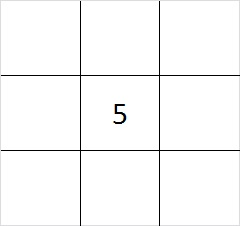Step 2: Put one of the even numbers in one of the corners.  You have four different choices, 2, 4, 6, or 8. The illustration is for the number 2, but any of the even numbers will work.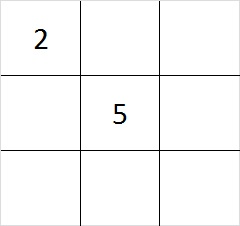Step 3: Subtract your even number from 10 to find its partner. 4 + 6 are partners and so are 2 + 8. Put the partner of the number you chose for step 1 in the corner that is diagonal to it.Step 4: Put the other two even numbers in the remaining corners. Yes, you have two choices where to put the numbers. Either choice will work.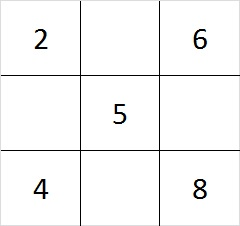Step 5: Since 6 + 8 = 14 and 15 – 14 = 1, put 1 in the cell between the 6 and the 8. Do similar addition and subtraction problems on each side of the square to determine where to place the 3, 7, and 9. You can work clockwise or counter clockwise, or skip around the square doing the addition and subtraction problems; it doesn’t matter.

This finished magic square looks like this: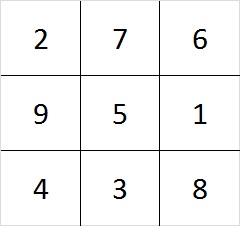Check it out! Every row, column, and diagonal adds up to 15!

As we created the square, we made choices. First we chose between 4 even numbers, and later we had 2 more choices. Notice that 4 x 2 = 8. There are 8 different ways to make a 3 x 3 magic square! (However, they are all really the same square turned upside down, rolled on its side, viewed from the back. etc.)

There are 880 different ways to make a 4 x 4 magic square. Look over the related articles at the end of this post to learn more about magic squares that are bigger than 3 x 3.

Speaking of magic squares, when I look at the square logic puzzle below, something magical happens. This puzzle has nine clues in it, and all of them are perfect squares. I can use those nine clues to construct a complete multiplication table. If you finish the same puzzle, your multiplication table will look exactly like mine because this puzzle has only one solution.The level 3 puzzle below is only a little bit more difficult. To solve it place the numbers 1 – 10 in the top row and again in the first column so that those placed numbers are the factors of the given clues. Again there is only one solution, and you will need to use logic to find it. Click 10 Factors 2014-01-06 for more puzzles and last week’s answers.May we all find a little bit more magic in our lives!